#### Moment-based approximations

Since the probabilistic I-states are functions, it seems natural to use function approximation methods to approximate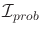. One possibility might be to use the first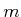coefficients of a Taylor series expansion. The derived I-space then becomes the space of possible Taylor coefficients. The quality of the approximation is improved asis increased, but also the dimension of the derived I-space rises.

Since we are working with probability density functions, it is generally preferable to use moments as approximations instead of Taylor series coefficients or other generic function approximation methods. The first and second moments are the familiar mean and covariance, respectively. These are preferable over other approximations because the mean and covariance exactly represent the Gaussian density, which is the most basic and fundamental density in probability theory. Thus, approximating the probabilistic I-space with first and second moments is equivalent to assuming that the resulting probability densities are always Gaussian. Such approximations are frequently made in practice because of the convenience of working with Gaussians. In general, higher order moments can be used to obtain higher quality approximations at the expense of more coefficients. Let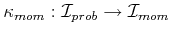denote a moment-based I-map.

The same issues arise for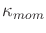as for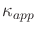. In most cases,is not a sufficient I-map. The moments are computed in the same way as the conservative approximations. The update equations (11.57) and (11.58) are applied for probabilistic I-states; however, after each step,is applied to the resulting probability density function. This traps the derived I-states in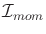. The moments could be computed after each of (11.57) and (11.58) or after both of them have been applied (different results may be obtained). The later case may be more difficult to compute, depending on the application.

First consider using the mean (first moment) to represent some probabilistic I-state,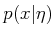. Let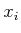denote theth coordinate of. The mean,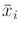, with respect tois generally defined as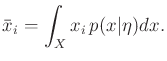(11.62)

This leads to the vector mean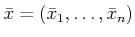. Suppose that we would like to constructusing only the mean. Since there is no information about the covariance of the density, working with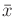is very similar to estimating the state. The mean value serves as the estimate, and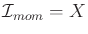. This certainly helps to simplify the I-space, but there is no way to infer the amount of uncertainty associated with the I-state. Was the probability mass concentrated greatly around, or was the density function very diffuse over?

Using second moments helps to alleviate this problem. The covariance with respect to two variables,and, is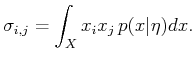(11.63)

Since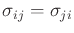, the second moments can be organized into a symmetric covariance matrix,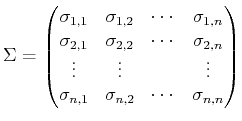(11.64)

for which there are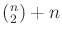unique elements, corresponding to every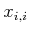and every way to pairwith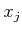for each distinctand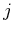such that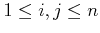. This implies that if first and second moments are used, then the dimension ofis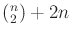. For some problems, it may turn out that all probabilistic I-states are indeed Gaussians. In this case, the mean and covariance exactly capture the probabilistic I-space. The I-map in this case is sufficient. This leads to a powerful tool called the Kalman filter, which is the subject of Section 11.6.1.

Higher quality approximations can be made by taking higher order moments. The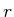th moment is defined as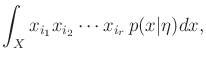(11.65)

in which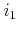,,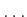,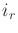areintegers chosen with replacement from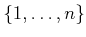.

The moment-based approximation is very similar to the conservative approximations for nondeterministic uncertainty. The use of mean and covariance appears very similar to using ellipsoids for the nondeterministic case. The level sets of a Gaussian density are ellipsoids. These level sets generalize the notion of confidence intervals to confidence ellipsoids, which provides a close connection between the nondeterministic and probabilistic cases. The domain of a Gaussian density is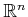, which is not bounded, contrary to the nondeterministic case. However, for a given confidence level, it can be approximated as a bounded set. For example, an elliptical region can be computed in which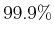of the probability mass falls. In general, it may be possible to combine the idea of moments and bounding volumes to construct a derived I-space for the probabilistic case. This could yield the guaranteed correctness of plans while also taking probabilities into account. Unfortunately, this would once again increase the dimension of the derived I-space.

Steven M LaValle 2020-08-14